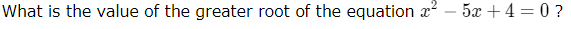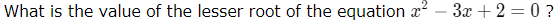This set of MCQs helps you brush up on important math topics and prepare you to dive into skill practice.

Start Quiz

Solve x^2 + 13x + 12 = 0 by factoring.

x = -12, 1

x = -12, -1

x = 12, -1

x = 12, 1

What are the 2 characteristics we look for when trying to solve a quadratic equation?

A positive coefficient for x^2x2 & the equation set equal to 0.

There is no such thing as a quadratic equation

Factor and cancel

Distribute and combine like terms

Determine the values of a, b, and c for the quadratic equation:
4x^2 – 8x = 3

a = 4, b =-8, c =3

a = 4, b =8, c =-3

a = 4, b =-8, c =-3

a = -4, b =-8, c =-3

Solve the equation by completing the square: x^2 - 8x = 20.

x = 10 and x = 2

x = -10 and x = -2

x = 10 and x = -2

x = 10 and x = 2

True or False: When factoring using the "bump it up" method, your FIRST step is to multiply the "1st" term ("a") and "3rd" ("c") term together. Then, look to see what factors of that product add together to get the middle "bx" term.

True

False5

4

1

-4

A standard form of a quadratic equation.

ax²+bx-c=0

ax²+bx+c=0

y=mx+b

y=mx1

2

-2

3

Quiz/Test Summary